# Introduction

I tried to solve `" Maximum average interval of daily visitors (equivalent to paiza rank B) "` from the skill check past problem collection (Java edition): blush: I'm new to programming, but I've summarized the algorithm part in an easy-to-understand manner! By the way, the author is B rank with no practical experience. ..

--Problem --Flow of how to solve --Answer code

# problem

### Problem statement

You were in control of a website. I ran a campaign on this website for a series of k days, but forgot how long it took.

Fortunately, we have access logs for all n days of running the website, and we know the number of visitors per day. For the time being, I decided to consider the period with the highest average number of visitors per day among the k consecutive days as a candidate for the period during which the campaign was conducted.

n days worth of visitors and campaign days k will be entered, so please output the number of candidates for the campaign period and the earliest start date among the candidates.

### Value to be entered

The input consists of two lines. In the first line, n and k are entered separated by a single-byte space. In the second line, n integers a_1, a_2,…, a_n are entered separated by a single-byte space. a_i represents the number of visitors on day i.

### Expected output

Please output the number of candidates for the campaign period and the earliest start date among the candidates in this order, separated by a half-width space on one line.

### Conditions

In all test cases, the following conditions are met. ・ 1 ≤ n ≤ 1,000 ・ 1 ≤ k ≤ n ・ 0 ≤ a_i ≤ 100

``````5 3
1 2 3 2 1
``````
``````1 2
``````
``````10 2
6 2 0 7 1 3 5 3 2 6
``````
``````5 1
``````

For details, please check ** here ** to check the problem!

# Flow of how to solve

### 1. Think about the general flow

I usually comment out and write the general flow before writing the code. This time I drew it in a diagram for easy understanding!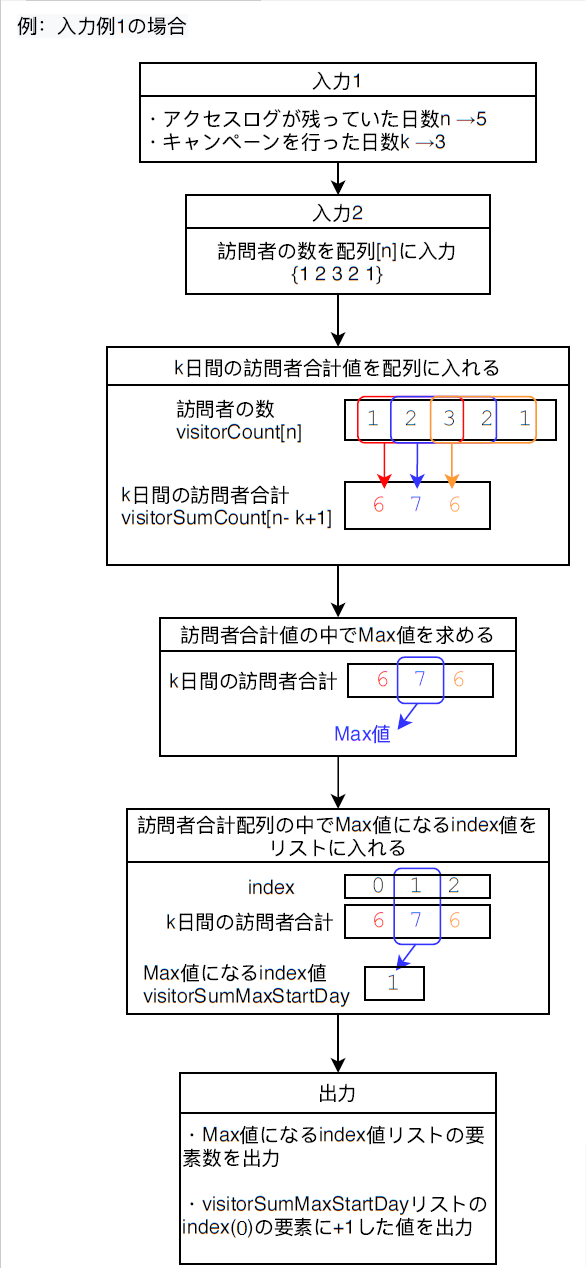### 2. Write the code

If you think about the general flow, I will write the code. I will write the code according to each process. I try to divide the parts where the scope is likely to be long into methods.

#### ① Input 1

The standard input uses the Scanner class.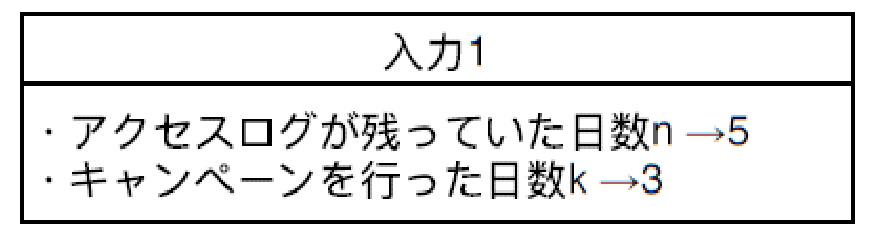`````` // Input
Scanner sc = new Scanner(System.in);
// Number of days the access log remained n
int n = sc.nextInt();
// Number of days the campaign was held k
int k = sc.nextInt();
``````

#### ② Input 2Divide into methods. First, write the initialization of the array and the call of the method. ↓

`````` // n-day visitor array
int[] visitorCount = new int[n];
// Enter the number of visitors (n days)
visitorCount = inputVisitor(n, visitorCount, sc);
``````

Next, I will write the contents of the method. ↓

``````	/**
* Method to enter the number of visitors (n days)
* @param n The number of days that the access log remained n
* @param visitorCount Array of visitors for n days
* @param sc Standard input
* @return Array with the number of visitors entered
*/
private static int[] inputVisitor(int n, int[] visitorCount, Scanner sc) {
for(int i = 0; i < n; i++) {
visitorCount[i] = sc.nextInt();
}
return visitorCount;
}
``````

#### ③ Make an array of total visitor values for k days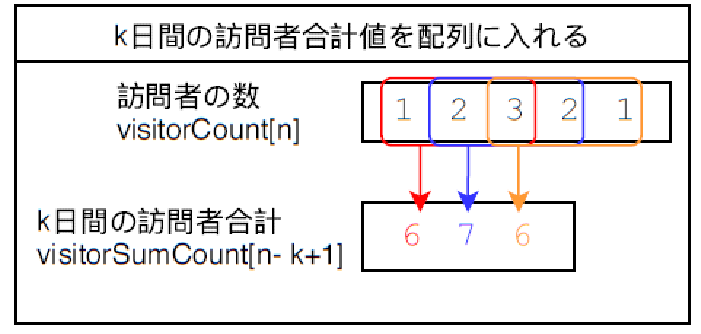Divide into methods. First, write the initialization of the array and the call of the method. ↓

`````` // Array of total number of visitors for k days [n --k + 1]
int[] visitorSumCount = new int[n - k + 1];
// Calculate visitorSumCount
visitorSumCount = visitorSum(visitorCount, n, k, visitorSumCount);
``````

Next, I will write the contents of the method. ↓

``````	/**
* Calculate the total number of visitors for k days ((n --k + 1) times)
* @param visitorCount Array of visitors for n days
* @param n The number of days that the access log remained n
* @param k Number of days the campaign was held k
* @param visitorSumCount An array of total visitors for k days
* @return An array that calculates the total number of visitors for k days
*/
private static int[] visitorSum(int[] visitorCount, int n, int k, int[] visitorSumCount) {
for(int i = 0; i < (n - k + 1); i++) {
for(int j = i; j < (k + i); j++) {
visitorSumCount[i] += visitorCount[j];
}
}
return visitorSumCount;
}
``````

#### ④ Find the Max value in the total number of visitors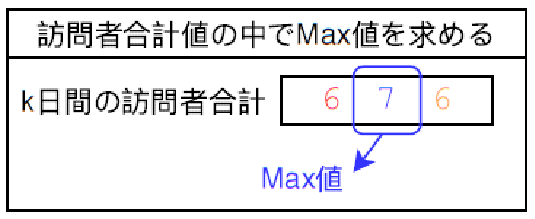Divide into methods. First, describe the initialization of variables and the call of methods. ↓

`````` // Max value in visitorSumCount
int visitorSumMax = 0;
visitorSumMax = sortVisitorSumMax(visitorSumCount, visitorSumMax);
``````

Next, I will write the contents of the method. ↓

``````	/**
* Find the Max value in the total number of visitors
* @param visitorSumCount An array of total visitors for k days
* @param visitorSumMax Max value in visitorSumCount
* @return Obtained Max value
*/
private static int sortVisitorSumMax(int[] visitorSumCount, int visitorSumMax) {
for(int i = 0; i < visitorSumCount.length; i++) {
if(visitorSumCount[i] > visitorSumMax) {
visitorSumMax = visitorSumCount[i];
}
}
return visitorSumMax;
}
``````

#### ⑤ Put the index value that becomes the Max value in the total visitor array in the list.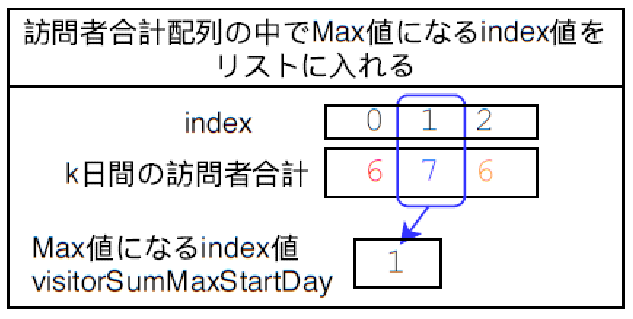`````` // List with index values that will be visitorSumMax
List<Integer> visitorSumMaxStartDay = new ArrayList<>();
visitorSumMaxStartDay = visitorSumMaxStartDaySort(visitorSumCount, visitorSumMax, visitorSumMaxStartDay);
``````

Next, I will write the contents of the method. ↓

``````	/**
* Save the index that becomes visitorSumMax in visitorSumCount in the list
* @param visitorSumCount An array of total visitors for k days
* @param visitorSumMax Max value in visitorSumCount
* @param visitorSumMaxStartDay Index that becomes visitorSumMax in visitorSumCount
* @return Returns an indexed list
*/
private static List<Integer> visitorSumMaxStartDaySort(int[] visitorSumCount, int visitorSumMax, List<Integer> visitorSumMaxStartDay) {
for(int i = 0; i < visitorSumCount.length; i++) {
if(visitorSumCount[i] == visitorSumMax) {
}
}
return visitorSumMaxStartDay;
}
``````

#### ⑥ Output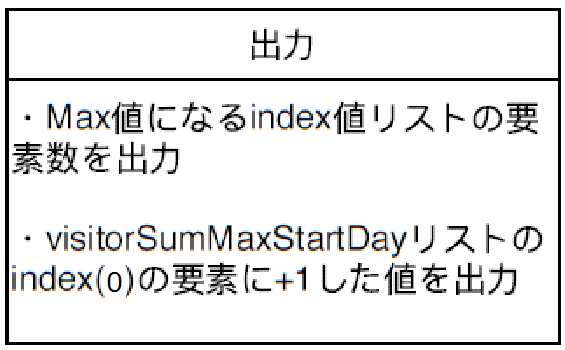This is the last! visitorSumMaxStartDay Outputs the number of elements in the list and the value of index (0) + 1.

`````` // Output the number of candidate days
System.out.print(visitorSumMaxStartDay.size() + " ");
// Output the first day of the candidate date
System.out.print(visitorSumMaxStartDay.get(0) + 1);
``````

Finally, I will post the answer code!

``````import java.util.*;

public class PaizaB01Visitors {

public static void main(String[] args) throws Exception {
Scanner sc = new Scanner(System.in);
// Number of days the access log remained n
int n = sc.nextInt();
// Number of days the campaign was held k
int k = sc.nextInt();

// n-day visitor array
int[] visitorCount = new int[n];
// Enter the number of visitors (n days)
visitorCount = inputVisitor(n, visitorCount, sc);

// Array of total number of visitors for k days [n --k + 1]
int[] visitorSumCount = new int[n - k + 1];
// Calculate visitorSumCount
visitorSumCount = visitorSum(visitorCount, n, k, visitorSumCount);

// Max value in visitorSumCount
int visitorSumMax = 0;
visitorSumMax = sortVisitorSumMax(visitorSumCount, visitorSumMax);

// List with index values that will be visitorSumMax
List<Integer> visitorSumMaxStartDay = new ArrayList<>();
visitorSumMaxStartDay = visitorSumMaxStartDaySort(visitorSumCount, visitorSumMax, visitorSumMaxStartDay);

// Output the number of candidate days
System.out.print(visitorSumMaxStartDay.size() + " ");
// Output the first day of the candidate date
System.out.print(visitorSumMaxStartDay.get(0) + 1);

sc.close();
}

/**
* Method to enter the number of visitors (n days)
* @param n The number of days that the access log remained n
* @param visitorCount Array of visitors for n days
* @param sc Standard input
* @return Array with the number of visitors entered
*/
private static int[] inputVisitor(int n, int[] visitorCount, Scanner sc) {
for(int i = 0; i < n; i++) {
visitorCount[i] = sc.nextInt();
}
return visitorCount;
}

/**
* Calculate the total number of visitors for k days ((n --k + 1) times)
* @param visitorCount Array of visitors for n days
* @param n The number of days that the access log remained n
* @param k Number of days the campaign was held k
* @param visitorSumCount An array of total visitors for k days
* @return An array that calculates the total number of visitors for k days
*/
private static int[] visitorSum(int[] visitorCount, int n, int k, int[] visitorSumCount) {
for(int i = 0; i < (n - k + 1); i++) {
for(int j = i; j < (k + i); j++) {
visitorSumCount[i] += visitorCount[j];
}
}
return visitorSumCount;
}

/**
* Find the Max value in the total number of visitors
* @param visitorSumCount An array of total visitors for k days
* @param visitorSumMax Max value in visitorSumCount
* @return Obtained Max value
*/
private static int sortVisitorSumMax(int[] visitorSumCount, int visitorSumMax) {
for(int i = 0; i < visitorSumCount.length; i++) {
if(visitorSumCount[i] > visitorSumMax) {
visitorSumMax = visitorSumCount[i];
}
}
return visitorSumMax;
}

/**
* Save the index that becomes visitorSumMax in visitorSumCount in the list
* @param visitorSumCount An array of total visitors for k days
* @param visitorSumMax Max value in visitorSumCount
* @param visitorSumMaxStartDay Index that becomes visitorSumMax in visitorSumCount
* @return Returns an indexed list
*/
private static List<Integer> visitorSumMaxStartDaySort(int[] visitorSumCount, int visitorSumMax, List<Integer> visitorSumMaxStartDay) {
for(int i = 0; i < visitorSumCount.length; i++) {
if(visitorSumCount[i] == visitorSumMax) {